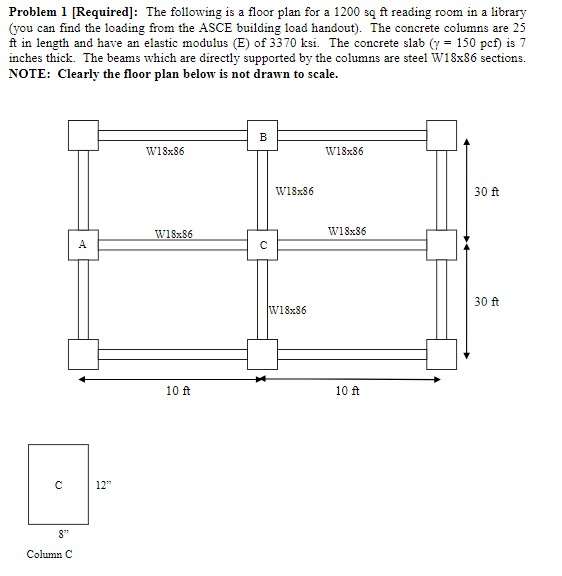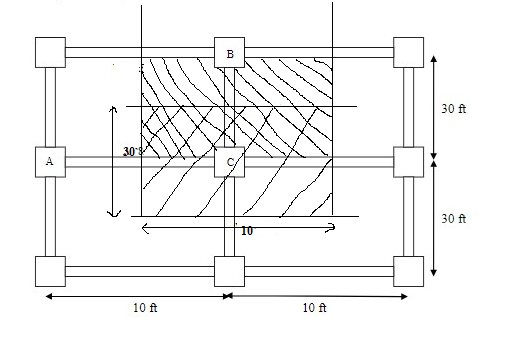# Problem 1 [Required]: The following is a floor plan for a 1200 są ft reading room in a library(you can find the loading from the ASCE building load handout). The concrete columns are 25ft in length and have an elastic modulus (E) of 3370 ksi. The concrete slab (y = 150 pcf) is 7inches thick. The beams which are directly supported by the columns are steel W18x86 sections.NOTE: Clearly the floor plan below is not drawn to scale.w18x86W18x86W18x8630 ftW18x86W18x8630 ftW18x8610 ft10 ft12"8"Column C

Question
10 views
The following is a floor plan for a 1200 sq ft reading room in a library (you can find the loading from the ASCE building load handout). The concrete columns are 25 ft in length and have an elastic modulus (E) of 3370 ksi. The concrete slab (γ = 150 pcf) is 7 inches thick. The beams which are directly supported by the columns are steel W18x86 sections. NOTE: Clearly the floor plan below is not drawn to scale.

(a) Does this system represent one way or two way slab loading?

(b) Calculate and draw the loading on beams A-C and B-C (include the self-weight of the beam)[answer in lbs/ft or kips/ft]

(c) Draw the shear and bending moment diagram of beams A-C and B-C (assuming simply supported end conditions). Label moment and shear at the ends and midspan of the beam.

(d) Calculate the loading demand on column C (neglect the self-weight of the columns) [answer in lbs or kips] NOTE: You can ignorethe reduced live load factor given in equation 1-1 ofthe textbook even though this column would satisfy KLLAT> 400 ft^2.

(e) Will column C buckle if the ends are pinned(k = 1.0)?

(f) If the deflection limits set forth by the ASCE building code are [in]less than L/360, does beam B-C pass? (note: Esteel= 29,000 ksi, Ix,beam= 1530 in^4)help_outlineImage TranscriptioncloseProblem 1 [Required]: The following is a floor plan for a 1200 są ft reading room in a library (you can find the loading from the ASCE building load handout). The concrete columns are 25 ft in length and have an elastic modulus (E) of 3370 ksi. The concrete slab (y = 150 pcf) is 7 inches thick. The beams which are directly supported by the columns are steel W18x86 sections. NOTE: Clearly the floor plan below is not drawn to scale. w18x86 W18x86 W18x86 30 ft W18x86 W18x86 30 ft W18x86 10 ft 10 ft 12" 8" Column C fullscreen
check_circle

Step 1

Note: As per honor code policy, we are authorized to answer up to 3 subparts at a time so we are answering the first three subparts. For remaining subparts please repost the question separately.

a)

To check whether the slab is one way or two way:

Where,

ly = length of long-span

lx = length of short span

if the ratio of longer span to shorter span is greater than 2. Then it will be designed as ONE WAY slab.

Step 2

b, c)

Because slab is one way along the short span and load is assumed to be carried by end beam

DA and BC.Step 3

SFD and BMD of beam AC:

Self-weight of beam = 0.086 kip

Calculation of shear force (Q):

Calculation of bending moment (M):
at the point x = 5:

...

### Want to see the full answer?

See Solution

#### Want to see this answer and more?

Solutions are written by subject experts who are available 24/7. Questions are typically answered within 1 hour.*

See Solution
*Response times may vary by subject and question.
Tagged in

### Civil Engineering